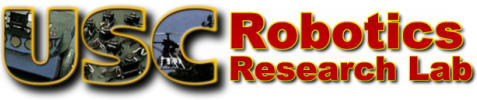Publication Details

. "Recursive Learning for Deformable Object Manipulation". Institute for Robotics and Intelligent Systems, IRIS-99-369, 1999. (.gz)

Abstract:

This research addresses the problem of robotic grasping of 3-D deformable objects. Specifically, we seek to develop a generalized approach for handling of 3-D deformable objects in which prior knowledge of object attributes is not required and thus can be applied to an infinite number of object types (e.g. asymmetric, nonhomogneeous, nonlinear object types). Our methodology relies on the implementation of two main tasks. Our first is to calculate deformation characteristics for a non-rigid object represented by a physically-based model. This model is derived from discretizing the object into a network of interconnected particles, springs, and damping elements. Using nonlinear partial differential equations, we model the particle motion of the deformable object in order to calculate the deformation characteristics. For our second task, we must calculate the the minimum force required to lift the deformable object. This lifting force consists of a combination of the base force required to lift a rigid object of the same weight plus an additional force term which successfully compensates for the deformation of the object. This minimum lifting force can be learned using a technique called iterative lifting. With this method, the robotic system learns the required lifting force by lifting the object with iterative measurements of force. Once the deformation characteristics and the associated lifting force term are determined, they are used to train a neural network for extracting the minimum force required for subsequent deformable object manipulation tasks. Our developed algorithm is validated with two sets of experiments. The first experimental results are derived from the implementation of the algorithm in a simulated environment. The second set involves a physical implementation of the technique whose outcome is compared with the simulation results to test the real world validity of the developed methodology. This real world implementation consists of a dual vision system and two cooperative manipulators, each possessing an end-effector constructed as a flat surface palm. Based on the simulation and real-world results, we are able to show that our physical, simulation, and theoretical lifting forces differ from each other maximally by a 14% error level.

HTML Reference:
<span class="author">Ayanna Howard</span>. "<span class="title">Recursive Learning for Deformable Object Manipulation</span>". <span class="institution">Institute for Robotics and Intelligent Systems</span>, <span class="number">IRIS-99-369</span>, <span class="month"></span> <span class="year">1999</span>.
Bibtex Reference:
@techreport{Howard-1999-322,
author = "Ayanna Howard",
title = "Recursive Learning for Deformable Object Manipulation",
institution = "Institute for Robotics and Intelligent Systems",
number = "IRIS-99-369",
year = "1999",
url = "http://robotics.usc.edu/publications/322/"
}
Affiliated Labs:

Other

Author Details:

Name:Ayanna Howard